September 28, 2006

scribe, transformation.Hi! I'm jessica. and I volunteered to do this scribe today since noone wanted too =/ Boo to you guys I guess. To start off with the morning class. Mr. K gave us questions on the board to do and here they are:
Given the graph of y=F(x) sketch:
A. y=-2F(x) B. y=F(-2x) C. y=-F(x/2) D. -1/2F(-x)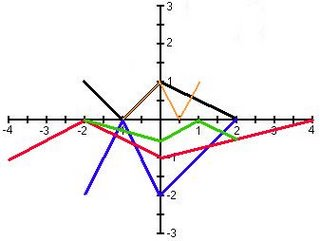For y=-2F(x), you multiply the y-coordinates by -2 (which will also produce a reflection over the x-axis). For y=F(-2x), you multiply the x-coordinates by 1/2 (which will also produce a reflection over the y-axis). For y=-F(x/2), you multiply the x-coordinates by 2 (which will also produce a reflection over the x-axis). And finally for -1/2F(-x), you multiply the y-coordinates by -2 (which will also produce a reflection over the x-axis) and you multiply the x-coordinates by -1 (which will produce a reflection over the y-axis).

If F(x)=x2-1, write the equation of:
A. y=F(x+2)
How you would do this is by taking the F(x+2) and substituting it into F(x) in the original function that was given to you.
Which would equal y=(x+2)2-1
B. y=F(1/2x)+1
For this one, you square everything in the brackets. Which would give you 1/4x2+1. Since it's a positive 1, it would cancel the negative 1 in the original function. Which will equal to y=1/4x2
C. y=-F(x-1)+2
What you would do is substitute the (x-1) into F(x). Which will give you (x-1)2+2. Don't forget the negative infront of the F, which will be distributed to (x-1)2 and to the negative 1 in the original function ( which will become a positive 1). Then it will all equal to y=-(x-1)2+3
D. y=2F(1-x)+3
How you would do this type of question is by substituting the (1-x) into F(x) in the original function. Then you would multiply 2 to the negative 1 in the original function (which will give you a negative 2). Then 3 subtract negative 2 is positive 1.
You would then get y=2(1-x)2+1
For some people, it may be confusing to get F(1-x). What you would do is factor the negative out. Which you would get is F[-(-1+x)] agreed? Okay, then just rearrange the x to be first. Which will look like.. F[-(x-1)].

Then Mr. K gave us F(2-x) to make an equation from F(x)=(x-1)3
You would do the samething as the above question. Factor out the negative, then rearrange the x.. Which you would get F[-(x-2)]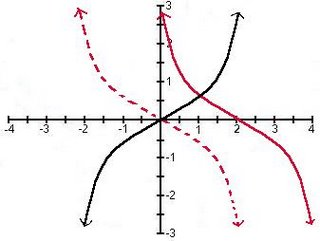The black cubic is the original graph. Then the graph was reflected over the y-axis (which is the dotted red line). Then you would shift the red line 2 units to the right.

In the afternoon class, Mr. K gave us notes to write in our math dictionaries.
Transformation:
Translations F(x-a)+b

The role of parameter a:
a>0 the graph shifts right a units.
- the x-coordinates are increased a units.
a<0 the graph shifts left a units.
- the x-coordinates are decreased a units.
WARNING: watch the sign of a

The role of parameter b:
b>0 the graph shifts up b units.
- the y-coordinates are increased b units.
b<0 the graph shifts down b units.
- the y-coordinates are decreased b units.

EXAMPLES: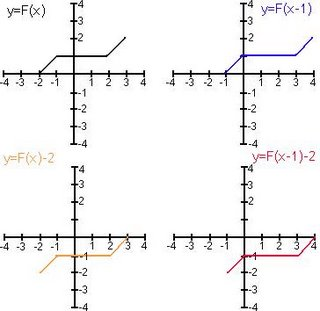Stretches and Compressions: aF(bx)

The role of parameter a:
a>1 the graph of F(x) is stretched vertically.
0<|a|<1 the graph of F(x) is compressed vertically.
- the y-coordinates of F are multiplied by a.

The role of parameter b:
b>1 the graph of F(x) is compressed horizontally.
(Everything "speeds up")
0<|b|<1 the graph of F(x) is stretched horizontally. (Everything "slows down")
- the x-coordinates are multiplied by 1/b.

EXAMPLES: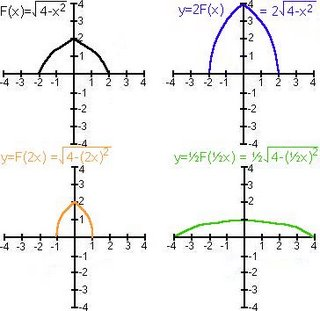Reflections:
Given any function F(x):
-F(x) produces a reflection in the x-axis.
- y-coordinates are multiplied by (-1)
F(-x) produces a reflection in the y-axis.
- x-coordinates are multiplied by (-1)

Inverses: the inverse of any function.
F(x) is F-1(x) (read as: "EFF INVERSE")
WARNING: F-1(x) does not equal to 1/F(x)
F-1(x) undoes whatever F did.

EXAMPLE: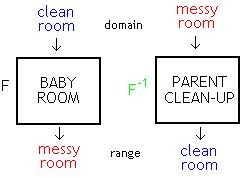This example is about Mr. K talking about his little girl. When she was younger, they had a play room for her to play in. They had blocks in a box that was all neat and tidy. They had another box of stuff animals that are neat and tidy. They had books of a shelf arrange from big to small, hard cover to soft cover. One day, Mr. K's little girl would go into the play room and mess everything up. Everything would end up scattered all over the floor. As it turns to night, Mr. and Mrs. K cleans up the play room. The following morning, his daughter would go back to the play room and mess everything up all over again. And when night comes, Mr. and Mrs. K cleans the room once again. Mr. K gave us an example of an inverse. Baby mess up clean room, then parents clean up messy room to become a clean room.
GET IT? GOT IT? GOOD (:

Inverses Considered Numerically:
As ordered pairs: given any ordered pair (a, b), it's inverse is (b, a).
As a table of values: given any function defined by a table of values in x and y. The inverse function has a table of valies where the inputs and outputs are exchanged: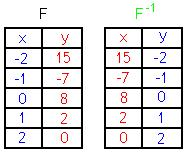Inverses Considered Symbolically (as equations):
method #1 - algebraically: given any function in x and y, to find the inverse exchange the x and y variables and solve for y.

EXAMPLE:
y=2x3-4
to find F-1..
x=2y3-4
x+4=2y3
x+4/2=y3
cube root x+4/2=y = F-1(x) (SORRY, i don't know how to put the cube root thingy for the whole thing =/).

method #2 - conceptually: given any function F to find the inverse list the operation applied to F, then rewrite them undoing each operation in reverse order.

EXAMPLE: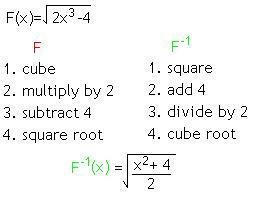Inverse Considered Graphically:
Given the graph of any function you can find the inverse by reflecting it in the line y=x.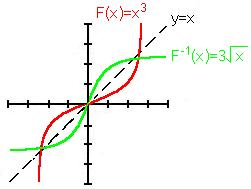Yeah, and that's about it.. Sorry if everything is all ugly/rushed. Because I pretty much just got off from work (10PM). Oh wells, at least I tried. Oh, btw.. the next scribe is cheeks (:

gooday & goodnight.

September 27, 2006

Scribe Post: Translations & Reflections

Hello everyone! I'm Jess and i'm the scribe for today's class. At the begining, we started with three questions about the transformations that we have learned yesterday. And here's the questions:
1. f(x) = x^2 ; Sketch f(x-3)+2

*As we know, -3 is C => shift to the right 3 units, and +2 is D (the last part of the function) => shift up 2 units, and we get the new vertex at (3,2)

2. The graph of g(x) is formed by sliding f(x) 4 units to the left. If f(x) = sin(x+2)-5 ; write the equation of g(x)

g(x) = sin(x+6)+5
*because f(x) moves 4 units to the left to get the graph of g(x), therefore it becomes 6
(x+2) = (x-(-2)) => (x-(-2)+(-4)) = (x-(-6)) => (x+6)

3. Below is the graph of y = f(x)
a. 2f(x) b. f(2x) c. 2f(x)-1
Here's all the graphs for the all the functions above:
a. for this question, we're doubling the y-coordinate
(-1 , 3) => 3(2) = 6 therefore we get the point (-1,6)
(-3 , -2) => (-2)(2) = -4 ; we get the point (-3,-4)
(4 , -1) => (-1)(2) = -2 ; we get the point (4,-2)
* in this one, 2 does not affect the x-coordinate
b. for this question, we're doubling the x-coordinate
(-1 , 3) => -1(1/2) = -1/2 therefore we get the point (-1/2,3)
(-3 , -2) => (-3)(1/2) = -3/2 ; we get the point (-3/2,-2)
(4 , -1) => (4)(1/2) = 2 ; we get the point (2,-1)
* in this one, 2 does not affect the x-coordinate
On the other note, in grade 12, we only deal with multiplication and addition, and we dont use division or subtraction.
Examples: instead of divide by 2, we multiply 1/2, and instead of subtract, we add the negative number of that number!
c. for this question, the function 2f(x)+1, both numbers affect the y-coordinate
(-1 , 3) => 3(2) = 6, then we shift 1 unit up => 6+1=7 ; therefore we get the point (-1,7)
(-3 , -2) => (-2)(2) = -4 ; shift 1 unit up => -4+1=-3 ; we get the point (-3,-3)
(4 , -1) => (-1)(2) = -2 ; shift 1 unit up => -2+1=-1 ; we get the point (4,-1)

Moving on, Mr. K started with a new function f(x) = sin(x)
if we change f(x) to -f(x), then we'll get -sin(x) => -f(x) = - sin(x)
if we change f(x) to f(-x), it will flip over the y-axis
from here, we come up with f(-x) = -f(x)
f(-x) = -f(x) This is called the definition of an odd function
The graph above is an odd function since if we flip it over the y-axis, we'll have a different graph.
- if we change g(x) to -g(x), instead of open up, the graph will open down
- if we change g(x) to g(-x), the function will flip over the y-axis, in this case, there's nothing change, the graph remains the same, from here, we come up with g(x) = g(-x)
g(x) = g(-x) This is call the definition of an even function.
*even function : no changes are made in the graphs

From those graphs above, we have 2 cases:
even function, g(x) = g(-x) and odd function, f(-x) = -f(x)
*Most functions are not odd nor even function.
Reflection:
- to reflect the x-axis, change the y-axis
- to reflect the y-axis, change the x-axis
Like Mr. K said, we still have one more type of transformation or function to talk about but since there wasn't enough time, so we'll leave it for tomorrow's class!
By the way, this is my first scribe post and it took me awhile to get this done, so hopefully, everyone understand it and if not, you know who you can look for help! And please do comment if i got anything wrong! Have a nice day everyone!
Oh and the next scribe post will be... japs_world. Good luck!

September 26, 2006

Scribe Post !

TRANSLATIONS

Alright, so I guess I'm back again, since TEST DAYS we aren't able to have a scribe, so here I am, and it is getting late. So first of all we started off the first class in the morning with .... The second part of our Unit Test from yesterday! Everyone was flabbergasted when Mr. K had mentioned the second part, and everyone was boo-ing.

So anyways, after the second part of the unit test, Mr. K solved the problem on the board. From that I saw all the mistakes I've done, and I felt like I just wanted to grab my test back and correct it, but what's done is done!

Soon after correcting those problems we went off to start the new unit. The new unit was called "Translations", which was familiar to some, and to some, it was new, and to the others, it was a review. Mr. K first wrote on the board and asked us what f(x) = x^2 was. I quickly raised my hand up and told him that it was something called a parabola, I have sketched it out on paint, and please don't laugh, it's just a rough sketch, well you may laugh if you'd like I won't get mad =), anyways the parabola I drew looked like this: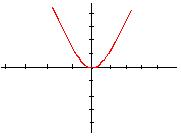Shortly after that, he continued on and asked us what f(x)= x^3 was and some of the class quickly said it's a cubic, some were probably muttering it quietly, and I yelled out, it looks sort of looks like an "S"! Mr. K, after showing us the cubic and parabola function, he then asked us what this function looked like f(x)= x. Some were quiet and pondering, while some were murmuring it out loud, and quickly I said, absolute value function ! Which looks like this: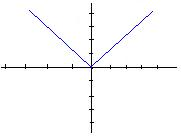Some people in the class when they heard about the absolute value function were stunned, because they had not heard of this function before, or maybe they've heard of it,but just forgotten. So a little explanation of an absolute value is basically, whatever is inside the absolute value brackets, tells how far away from zero you are. For example: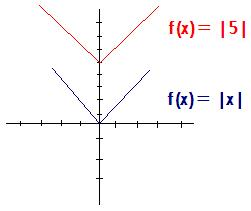Basically what happened in that graph is instead of "x" it was changed into "5" and that indicated how far away from zero you were, hence that is an example of the definition of the absolute function.

Mr. K then returned us to the basic sin graph and he showed to us the relation to between the graphs. Mr. K asked, "Are these two graphs the same?" The class looked puzzled and we answered no they don't "look" the same. Mr. K replied "That's right, but their equations are the same." " If you know what the basic graph looks like and someone adds something to it, then you'll know what the graph will look like, even though someone added something to it," said Mr. K.

Before we ended the first class, Mr. Kuropatwa asked us, "Do you know what sliding a function is called?" The class looked at him with confusion. Then he tried to show us many hints but we did not get it, until he finally gave up and told us that it was called a translation, he had asked us if we've heard of that term before and I believe some of the class answered "yes", i for one said yes, because it was all coming back, well most of it was coming back.

For the second class, Mr. Kuropatwa had left some questions for us to do. One of them were:

2. If (-2,4) is a point on the graph of y=f(x-1) what must be a point on:

a) f(x)+2

b) f(x+2)

c) f(x-3)-1

Now when you read that question normally you think you got that all figured out, am I correct? Well you haven't figured it all out, since (-2,4) is a point on the graph, you still have not yet finished! What you may have failed to do was to find the original or basic graph f(x). In this case f(x) was (-3,4) how did we know this? Well the question pointed out that (-2,4) was only a point on the graph y= f(x-1). But to arrive to our basic graph we simply just took -2 and -1 and it equaled to -3 for the x value. Now that we have our original graph we are all set to solve those questions.

After we done those questions we played a little game, well, it wasn't really a "little game", more like playing with our calculators. Mr. K showed us a few things about the circle and it not being a function, because it doesn't pass the vertical line test, which at first many of the people in the class did not remember.

Lastly, Mr. K told us to do some of our homework from exercise 7, and 8, and left the class. I hope what I have written today will be helpful, and I would like to do this again another time, so until next time keep reading your notes before you go to bed, and ask many many questions during class so that it may benefit you by growing and learning! Take care to you all!

Also the word of the day!

FLUMOUX - It means to surprise you. It's a very great word.

Next Scribe is Jess. ( sorry for the mishap, it turns out that there are no scribes during test days, so for tomorrow you will be scribe! have fun =) . )

September 25, 2006

Gerald's blogging on blogging

Right now I think I am doing pretty bad. I understand it one second then I get lost in the next second. When Mr. K puts questions on the board, I am clued out half the time. After he explains it, I understand but the next set of questions I'm lost again. I think I'll find a tutor or something because I need a better understanding of things without holding back the class. I think I must come during my spare and get a better understanding. Above all, I love the way Mr. K teaches, he actually becomes part of the class rather than just being in front of the class. Understand me? hopefully...because sometimes I don't understand myself. =)

Lessons from the Geese?

The geese who inhabit the wildlife preserve where we walk each day have hunkered down away from humans, preparing I think for their migration. Occasional small formations of geese fly to and from the preserve these days, but theydon’t come looking for food from us as they did in spring. I wonder if you see them, too, heading south for the winter flying along in "V" formation? Do you know what science has discovered as to why they fly that
way?

FACT 1 - As each Goose flaps its wings it creates uplift for the birds that follow. By flying in a V formation, the whole flock adds 71 per cent greater flying range than if each bird flew alone.

FACT 2 - When a Goose falls out of formation, it suddenly feels the drag and resistance of flying alone. It quickly moves back into formation to take advantage of the lifting power of the bird immediately in front of it.

FACT 3 - When the lead Goose tires, it rotates back into the formation and another Goose flies to the point position.

FACT 4 - The Geese flying in formation honk to encourage those up front to keep up their speed.

FACT 5 - When a Goose gets sick, wounded or shot down, two Geese drop out of formation and follow it down to help and protect it. They stay with it until it dies or is able to fly again. Then, they launch out with another formation or catch up with the flock.

Are there lessons we can learn from a gaggle of geese? What do you
think?

I think Oliver's comment is right on target! Teamwork is one of the best ways to achieve.

I'm adding the lessons from the geese I've found to be true; they mirror Oliver's thoughts and perhaps add some food for thought.

FACT 1- As each Goose flaps its wings it creates uplift for the birds that follow. By flying in a V formation, the whole flock adds 71 per cent greater flying range than if each bird flew alone.
LESSON - People who share a common direction and sense of community can get where they are going more quickly and easily because they are traveling on the thrust of one another.

FACT 2 - When a Goose falls out of formation, it suddenly feels the drag and resistance of flying alone. It quickly moves back into formation to take advantage of the lifting power of the bird immediately in front of it.
LESSON - If we are as smart as a Goose we stay in formation with those headed where we want to go. We are willing to accept their help and give our help to others.

FACT 3 - When the lead Goose tires, it rotates back into the formation and another Goose flies to the point position.
LESSON - It pays to take turns doing the hard tasks and sharing leadership. As with Geese, people are interdependent on each other's skills, capabilities and unique arrangements of gifts, talents or resources.

FACT 4 - The Geese flying in formation honk to encourage those up front to keep up their speed.
LESSON - We need to make sure our honking is encouraging. In groups where there is encouragement, the production is much greater. The power of encouragement (to stand by one's heart or core values and encourage the heart and core of other) is the quality of honking we seek.

FACT 5 - When a Goose gets sick, wounded or shot down, two Geese drop out of formation and follow it down to help and protect it. They stay with it until it dies or is able to fly again. Then, they launch out with another formation or catch up with the flock.
LESSON - If we have the wisdom of Geese, we will stand by each other in difficult times as well as when we are strong.

Do you see any value in these lessons?

Scribe Post

Well folks, I'm the scribe today, but the only problem is, Mr. K was not available in our class today because well I'm not even sure, my only guess is that he's probably sick since he's been coughing and sneezing during school, so at least he's gotten some rest for today. I'm not sure what to put here because we did have a substitute for today and only had one class for the day which makes it difficult to write anything. Although we did have this test today and I found the test in the middle of easy and difficult. Although personally I believe I understood most of what was going on in the test, and hopefully I had done well. A few questions on the test did bug me, because I had not known what to do on it, one of the questions were determining the period, and this other question about a point on the unit circle and some kind of point that made 3.45 rotations, plus I also had a bit of trouble on the last question, I tried to do the best I can on them but have forgotten on what to do with them. Other than that, I think I did okay, and this is all for now until next time.

I will choose Jess as the next scribe, but if this scribe post isn't good enough since we had a substitute I'd be more than happier to make another one for tomorrow's class.

Jess is next scribe.

Jessica.'s Blogging on Blogging.

Hi, this is my first blogging on blogging. So far what we have learned is pretty straight forward. I'm getting everything that we're learning. I'm not having any difficulties with the unit circle or the new unit that we're starting. Well if I do, I will ask for help (:

Patrick's Blogging on Blogging

hi people! this is my blogging on blogging. things are good great i guess....this is my second year in Pre-Cal (haha) i am not having any trouble in unit circle and the new unit we did about graphing, but sometimes some of the question takes me longer for me to answer than the other questions, I have to read or think about the question 2 times sometimes in-order to answer correctly but i still understand the whole thing

September 24, 2006

Tennyson's Blogging on Blogging

Well i already taken this course once, but this time it's different. The teaching method is different from the last school that i came from. I guess last year i didn't study and pay attention in class as much, and was afraid to ask questions. This year I'm trying to improve that by taking your idea of studying everyday for a couple of minutes, and review my notes. The other thing that have to try doing is study earlier , not last min. I really like the fact that you try to make the students understand the question before moving on to the next question. Well that all i have to say , i hope i pass the test , this unit before was like one of my weakness. by3

Jess' Blogging on Blogging

Hi everyone! :) This is my first blogging on blogging! Well, to start this off, all i can say is "Pre-cal is errr.." even though it is my favorite subject! It is really getting harder each day, and I'm just afraid that i'll get lost in the few weeks, but hopefully not. Anyways, all the examples and questions that we usually use to start off the class are really help because i think it brings back the stuffs that we learned the day before so we wouldn't forget how to this and that, plus, we also learn new stuffs from it, so i hope that Mr.K would give us more and more practices like this! Well, i guess that is it. It's kinda late now and i have to review the notes, and all those examples, so goodluck on the test everyone! :)

Steve's Blogging on Blogging

Greetings, this is my first post on this blog. We're 3 weeks into the school year and our first test happens to be tomorrow, great. >_>; Doing some last minute studying i remember i have to write this thing for that oh so needed mark on the test. Well from the start i had some (i say some) experience with circular functions, so all that memorizing the unit circle and all the fixin's came from digging it all out from the o' noodle. Like other fellow students have mentioned in their posts, yes it is getting tougher, and Mr.K is taking it up a notch week after week, with that said the pre test was horrible to me ;_;. Learning from my mistakes (knowing "try try again" is officially a taboo as of a few days ago) hopefully i am able to keep up the pace. Well, this ends my post. Cheers to a hopeful successful semester.

-ScubaSteve

ruschev's Blogging on Blogging

hi everybody! this is my blogging on blogging. well....so far so good i guess....i am not having any trouble in unit circle and the new unit we did about graphing, but sometimes some of the question took longer for me to answer than the other questions, I have to read the the question 2-3 times sometimes in-order to figure out what to do then after that KABLAM!! (answered the question). If i were to give my self a percent on where i am right now on our math class.....i am giving my self 7 fried chicken wings out of 10

daphne's blogging on blogging

This is my first blogging on blogging...Well mathematics is one of my weakness when it comes to academic subjects. I really found it hard to understand in just a snap the topics discussed in this subject. It requires me to focus more on each of the topics in order for me to understand it well. In our class, I found Mr. K helpful because he explains the lessons well. Sometimes he gives some examples applied in our daily living for us to understand it more. Moreover, some of my classmates help me in some of my problems which regards in solving mathematical problems.
Math lessons are hard to understand but very helpful when you get it. One time during our period, I asked Mr. K a question that pushed him to explain the pattern I can't understand. He taught me the easiest way to memorize the exact values in the unit circle and when I got it, it helped me to have high scores in our mental math quiz. I find it very cool because I didn't see the pattern on the first place. As a conclusion, Mr. K teaches us in a very understandable way of learning math. All I can say is I have to work harder in order to achieve that high grade.

Richard's Blogging on Blogging

Hi! This is my first blogging on blogging! At first, the lesson was easy(should I say that it's easy?). As the lesson goes on, it seems like it's getting a bit difficult. I think the lectures are discussed so fast. My brain can't handle them all. Though I understand Mr.K's side that he must discuss all the the topic in the course outline before the end of the semester. I hope he give more examples for our better understanding. As of this moment, I can say that I've learned a lot from this class and I'd like to thank to Mr.K for that. We took a pre-test last meeting and it was different to what we had discussed. But the SPY TIME was really great!
That's it for now.. I hope everyone of us will pass in the test. Good Luck!

Allens' Blogging on Blogging

Okay what should I say first......... Ah! I know, math is hard. What else?......... yeah that's about it. Well I didn't think PreCal would be a walk in the park, I completely understood what we where tough in the first few classes, but the trig stuff not so. Last year I was pro when it came to the circle stuff, but it didn't have trig involved. Once we started the trig unit I was basically completely focused on learning it but then I kinda dosed off for a second and BAM! I was lost. But I am starting to catch up, after re-reading my notes over and over again, and also visiting the Scribe posts, that are really helpful. Also the circle stuff is actually interesting, like how sin and cos are all related in some way. And um...................where was I? Ah, yes that's it I guess.
Oh one more thing does my writing have to be green?

-AllenAssume this clock is mathematically correct, i.e. 0 is at the right and the hands move around counterclockwise ... What time is it in radian hours and minutes? ;-)

Some Reviews
What's the connection between circles and triangles? That's what we've been talking about in class.

Trigonometry has something to do with it. I found an excellent site that allows you to play and learn all about it.

I found a really neat site that illustrates the geometric interpretation of sine, cosine, tangent and cotangent that we've talked about in class. Check it out! You can change the lengths of the lines in the figure by clicking on the point on the circle where the "sin x" and "cos x" lengths intersect.

You can do a little review here about how to graph the sine, cosine and tangent functions.

And finally here is where you can find some excellent interactive review exercises. Try Topics #30, 31, 32 and 34. Omit Topic #33; we'll be covering that material in our next unit.

Learn Hard!! ;-)

September 23, 2006

scribe post

Hey it's Anh, your scribe for friday...hmm I just want to say that blogs are awsome but VERY frustrating. This scribe post took forever......='( stupid htmls.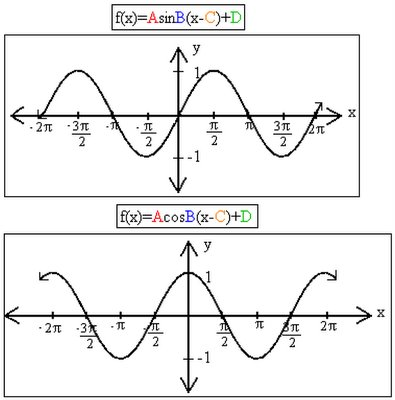The role of parameter A

IAI is called the AMPLITUDE.

"A" determines the distance of the maximum and the minimum values from the sinusoidal axis(the average value).

IAI > 1 The graph is stretched vertically.

0<IAI<1>A<>sinusoidal axis.

The role of parameter B

B determines the period of the function according to the equation.

P = 2π/B or B = 2π/period

NOTE: B is NOT the period; it determines the period.

The "period" is the distance along the x-axis required to make one "wave" of the function.

The role of parameter C

C is called the PHASE SHIFT AKA the HORIZONTAL SHIFT.

C > 0 The graph shifts right C units.

C < 0 The graph shifts left C units.

WARNING: watch the sign of C.

The role of parameter D

D is called the VERTICAL SHIFT AKA the AVERAGE VALUE of the function.

D determines the SINUSOIDAL AXIS.

D > 0 the graph shifts up D units.

D < 0 the graph shifts down D units.

The relationship between sine and cosine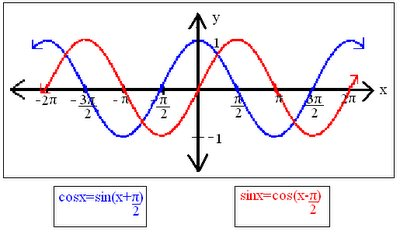They are related by a phase shift of Л/2.

Properties of the 3 basic trig functions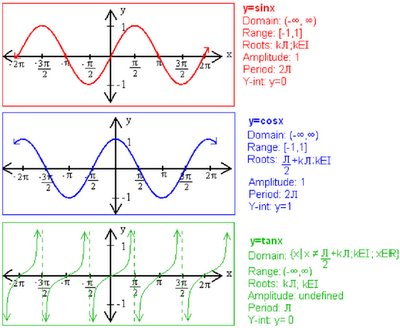Oliver's Blogging on Blogging

Ok, so this is my Blogging on blogging and I would just like to say that his math class is great, Mr. K is a good teacher, who speaks in depth and so far I some of it, is understandable to me. I'd like to say that what we did on fridy was a good start on forming some study groups, and also good way to get acquainted with some of our classmates, because you know we usually just sit on one part of the classroom and never really get to go to the other side. Also, when I was in calgary, we had like tutorials in the morning, when the teacher would come early, like around 8:00 am, and we would get to ask for questions we couldn't do on homeworks , or we get to see some of our old tests and ask how to figure them out, and I believe it was very helpful for me last year, and maybe that can happen here too, well it doesn't have to happen, just a thought I guess. Wel this is it for my blogging on blogging, Oliver B signing off. * Click *.

JessicaJill's Blogging on Blogging

Hello world! So, we're done with the unit circle and ready for the test. Am I preprared? I'm pretty sure I am, I just have to watch out for little tricks here and there with certain questions and make sure I know what they're asking me for. The unit circle is easy to remember, thanks to Mr. K's comprehendable teaching methods. I don't really have much else to say except good luck to everyone.

Lisa's Blogging on Blogging

Well.. well.. well.. what to say?? Um ok, so Manitoba is so much different than Ontario... So back home our grade 11 course sort of resembles this pre-cal course... we learned radians, and sine and cosine curves, but never in so much detail... math has never exactly been my favorite or best subject, and when i took my grade 11 course I didn't exactly understand a lot of what the teacher was saying. She didn't explain why radians are what they are, or many of the other things we've learned in this class, so this is like learning everything you have to know after already learning it the hard way.. ugh!! Unfortunatly even though this is like my second time taking the course, I'm still struggling with it... for example, in my school we never learned almost anything about the circle.. yesterday when Mr.K asked us what a tangent function looked like... and everyone was like "Oh ya" after he showed us, I still was like "What??" not knowing some of the basics that you guys have already learned is slowing me up... I'm learning them... but it doesn't seem quickly enough... I guess i'm a little slow in this department :P all i can do is work hard, ask about what i don't understand (even though I'm a little shy) and try my best... well i guess we'll see how i do on the next test :P .... Well i guess that's all for now....

September 22, 2006

James' Blogging on Blogging

Wow, I didn't know we had to do this... Anyways this is my first post on the blog, I came into the classroom expecting just as much since i've been in precal 40s already. But the teaching method is completely different from what I have had last year, and you know, I like it. I already have seen this stuff so it's nothing new, but I am still learning new things and RE-learning the things I have forgotten over the summer. I like how we have a math dictionary, last year I had a million stacks of paper, no idea where to start. Umm... Yeah I guess that is it!

- James

September 21, 2006

Ashley's Blogging on Blogging

Okay so this is my first blogging on blogging post and what can I say?! *thinks hard* Oh I know!! Math is hard!!! C'mon admit it is. I remember vaguely on the first day of class I was completely lost because Mr.K was talking about circles and radians and degrees and angles. I look around the class and it seems like most people get it and I'm like 'huh'?! But after a few classes more i finally get the whole radians thing on the circle. like how to plot the radians on the circle. for awhile there i had no idea what 3Π/2 was but after close examination i finally got it and i was so happy! a week later my happiness died and i became confused once again after Mr. K taught us about graphing trigonometric functions. i sort of get it now after asking my fellow classmates for help. my progress in class i suppose you could say is like a turtle walking. I'm slowly understanding. it just takes time for my brain to process everything Mr.K says when he talks. hopefully I'll do alright on the test*crosses fingers*

My Blogging on Blogging

This is my first Blogging on Blogging for our first unit before the test. I really learned a lot from the beginning of the year. At first when Mr. K started teaching us the values on the unit circle and the exact values, and how to find them, it was pretty confusing. But eventually everything started coming together. I just have to remember how to apply the equations that were written in our math dictionary and apply them to the problems Mr. K will be giving on the test. I just hope I'll remember the little things so I don't lose half marks. Let's just hope I do well on the test!!

Scribe Post _Graphing Trigometric Functions_

Hi, I’m Allen, and I am the scribe for today.
Today we just extended our knowledge about
how to graph trigonometric functions.
Mr. K started the class off with asking us
to graph the following trigonometric functions:

1) y=sin2x-1
2) y=2cosx+1
3) y=2sin(Πx)

~*Solutions:*~
1) y=sin2x-1

2) y=-2cosx+1
Then we had to graph 2 trigonometric functions with a
phase shift. The questions are as follows:

1) y=sin(x-(Π/4))
2) y=-cos3(x+(Π/6))

~*Solutions:*~
*The way you would graph a phase shift function is to first
draw the graph as if there was no phase shift. So the function
would look like this " y=sinx". Now since there is a -(Π/4) in
brackets beside x the entire graph will move right, NOT left
as the negative (Π/4) suggests.*

1) y=sin(x-(Π/4))
2) y=-cos3(x+(Π/6))
After that we where given a graph and was asked to give at
least 1 cos and 1 sin equation that would results in the
following graph:
~*Solutions:*~
The easiest way to solve a problem like this is to find the values
in the formula "A sin B (x-C)+D" so A, B,C and D. But first you
must choose a point to start from.

-Example1: for sin if I chose the spot where the line intersects
the y axis.-
A=2 because the graph shows the min and max is 2 units up and
2 units down.
B=4 because the graph shows that there are 4 waves in 2Π
(you find this by using the formula "period=(2Π)/B" and if
you put 4 in the result is Π/2 which is the end of 1 wave.
C=Π/8 because this is the phase shift the graph would
undergo to result in its current form if I was looking at it as
a sine value.
D=1 because the horizontal plain where the min and max are
equal occur at positive 1 on the y axis.

So the Final answer would be:
2sin4(x-(Π/8))+1

-Example2:for cos if I chose the spot where the line intersects
the y axis.-
A=-2 because the wave starts from its min where it intersects
the y axis. Which is down 2 from the horizontal plain where the
min and max are.
B=4 because the graph shows that there are 4 waves in 2Π
(you find this by using the formula "period=(2Π)/B" and if
you put 4 in the result is Π/2 which is the end of 1 wave.
C=0 because I chose the point where the line intersects the y
axis. Which happens to be the min so no phase shift has occured
(only in the way I choose) ,resulting in a normal cos function.
D=1 because because the horizontal plain where the min and max are
equal occur at positive 1 on the y axis.

So the Final answer would be:
y=-2cos4x+1

*Note* that these are only 2 out of a possible
because each point where the wave reaches i
ts max or min has its own set of A,B,C,&D
combos and since the wave keeps going and
going ( Ill stop there because I may get fined
or something for copying energizers saying.),
but all the equations would be the same becuase they
are on the same wave!!.

Thats all for now, and now for the
moment you've been waiting for
the next scribe will be Anh!!!
And my brother choose this name
so dont get mad at me!

September 20, 2006

I know this class should be fairly similar to me but honestly I feel like I'm learning it all for the first time. I can say truthfully that I'm understanding everything so far. The sine and cosine functions must be my favorite unit =). The only thing that had me a little confused was the triangle in the unit circle questions. The quadrants you draw them in, but I think most people are having trouble on that as I observed from the quiz. I think I figured it out and hopefully I'll get the question right on the test. Everything is good now but just wait till the unit on logs...I'll be lost.

Jho-Ann's blogging on blogging

This will be the first post from me on this blogging on blogging =). When we first came to class, we were asked why there was 360 degrees in a circle, of course i did some research and my results were because in ancient times, they used a base of 60 , when we now use a base of 10, but we went further on and discussed another reason why there was 360 degrees in a circle. We began to learn all these values within a 360 degree circle. For ex. sin of 90degrees = 1, then therefore led us into the unit we were about to learn; the unit circle/ circular functions. At my previous school I have already learnt the unit circle/circular functions, but it wasn't explained as well as how Mr. K explains it. He explains in detail on why those values became to be those values, i really like on how he goes in depth,he makes it easier for me to understand the concept.I believe that I'm progressing in his class in comparison to last year, where i was pretty much guessing every value on the unit circle, whereas of now i don't have to guess, because i KNOW it. It's amazing actually because you'd think there are so many values to remember but actually there's not,you'll notice the patterns, as what Mr.K repeats over and over in class is that," Math is the science of patterns". We just have to look for them. Hm mm..a brief way to compare and contrast DEGREES VS. RADIANS...dun dun dun...sounds hard?! (laughing) just kidding..well it is actually a little hard to explain. The only similarity that i can think of as of now is that they are both units of measurement, the difference between the two units of measurement is that degrees has a little circle symbol following after the number, so we know its a form of measurement in degrees, whereas radians has no symbol following after its number, therefore we must automatically assume its measured in radians.

Blogging

As far as the classes go I'm not having too many problems, just the usual confusions involving how an equation was solved and all. Progress has been slow but that's mainly due to the fact that I must have forgotten almost 80% of what I've learned over the summer break. Thankfully I finally have a grasp of the unit circle, not a good one but still it's a start at least. Just too bad that the whole understanding kinda just comes and goes, one minutes it all becomes clear to me and the next gone like the wind, I can only pray that it won't be happening during the test.

jefferson's blogging on blogging

Well this will be my first blogging on blogging before the test on Friday. Well, what can i say? Things are going smoothly. I've understands things enough to do exercises with ease. I almost know the whole unit circle and all i need is just to remember some tangent values. Convertions is like walking in a park. Finding points on a unit circle is like a piece of cake. And everything else is like CAKE WALK! But i still think I need to review my notes over and over just to sharpen my memory.

September 18, 2006

Scribe Post

(sine,cosine,tangent in unit circle)

hey hey! it is I ruschev, and i am the 7th scribe for today.LETS GET IT ON! shall we?

At the beginning of the class, Mr.K gave us this questions.

a. (Cos phi over 6) (Sec phi over 3) - (Tan negative phi over 4)

*Note Secavt (sec) is the reciprocal of cosine.
Example: if the answer in cosine is ( phi over 3 ) then the Sec will be ( 3 over phi ). just flip it.

I'll first start from Cosine to tangent.

we know that cosine is the x-axis and sine theta is the y-axis on the graph and phi over 6 is a radian so if you convert radian into degrees it will be 30 degrees (using converting degree and radian mr.k gave us)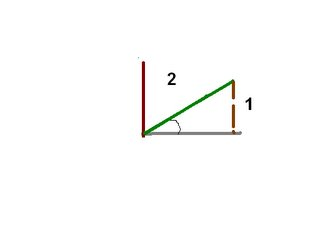heres a little drawing that i made, it is a 30 degrees and in radian this is phi over 6.

we are looking for the (cos phi over 6)

this simply means that we are looking for the

X coordinate of phi over 6 ( 30 degrees).

but how can we find the X coordiante of phi over 6?

we do the A squared + B squared = hyp squared

formula.

1 squared + B squared = 2 squared

1 + B squared = 4

( move 1 on the other side which will change it sign into + into - )

b squared = 3

( to remove the squared we have to put root sign on both sides)

so B will = root 3

so root 3 is the Adj. Now we can find sine and cosine.

sine is opp over hyp = 1/2

cosine is adj over hyp = root 3 over 2

And the question ask us, what is the cos of phi over 6? oh! it is root 3 over 2!

the next is (sec phi over 3 ).

oh wait ! sec is the reciprocal of cosine! i can just pretend that this is (cos phi over 3) and just flip the answer.

this is pretty much the same what we did earlier.

the only difference is that we have to find the opp side.

so we do the X squared + 1 squared = 2 squared again.

after doing some caculating...the opposite side should be root 3.

now we can find sine and cosine.

sine (y-axis) = root 3 over 2

cosine (x-axis) = 1 over 2

Oh! wait! the question ask us what is (sec phi over 3) cosine is 1 over 2, then the reciprocal of cosine is 2 over 1 or just 2, so the answer for (sec phi over 3 ) must be 2!

(tan negative phi over 4)

We know that tangent is sine over cosine.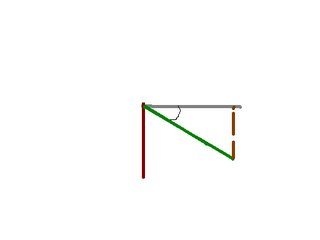since it is negative your diagram should be also at negative.

all we have to do on this part of the question is to dived sine and cosine.

lets say (phi over 4) is at 45 degrees, and you dont know what the opposite and adj. all you know is the hpy. hyp is 2.

all you have to do is use the formula x squared + x squared = hyp squared again.

x squared + x squared = 2 squared
2x squared = 4
dived both side by 2 because you want to get X by it self
x squared = 2
get rid off the squared by putting root on both sides
X = root 2

so opp = root 2, adj = root 2

now you know all sides!

sine = root 2 over 2
cosine = root 2 over 2

tangent = sine over cosine
tangent = (root 2 over 2) over (root 2 over 2)
tangent = 1

WAIT!!! it says tan(NEGATIVE phi over 4), so the answer must be a negative!

hmmm.. the answer is 1 but its a negative.....oh! it must be -1!!

now to multiply all the answers.

we have (root 3 over 2) (2) -(-1)
the 2's reduced and the final answer is (root 3 +1)

Then Mr.K kicked the question up a knocth!

he gave us

(2cos squared X - cosX=1)

fisrt. you move 1 on the left side making it negative

(2cos squared X - cosX-1 = 0)

then you have to factor it.

(2cosX+1) (cosX-1) = 0

you can check your factor to see if it is rigth by multiplying it.
you have to first multiply (2cosX and cosX) = 2cos squared X
then multiply (2cosX and -1) = -2cosX
then multiply (cosX and 1) = cosX
then multply (1 from -1) = -1
so you have (2cos squared X -2cosX + cosX -1) = 0
the -2cosX + cosX will be -cosX
(2cos squared X -cosX -1 = 0) (correct)

Now i know my factor is correct.

(2cosX+1) (cosX-1)

for the (2 cosX+1) we have to dived by 2 to get rid off the 2 on the front of cosX, so it will be
cosX= -1/2 (it is negative because i moved it to the other side)

we do the same thing for (cosX-1). cosX=1

Now to find the X.

CosX=-1/2 , this means that we are looking for all the x-axis that has -1/2.
CosX=1 , this means that we are looking for all the x-axis that has 1.

X= (2phi over 3) , (4 phi over 3) , zero degree , and (2 phi) .this are all the radians that have cosX=-1/2 and 1

That's all for now!!!

THE NEXT SCRIBE SHALL BE japs_world!!!!!As we discussed in class today, over the course of the semester we'll be publishing work to the blog and working collaboratively in our learning. We'll all need to use word processors and spreadsheets and other software. In today's connected learning enviroment we can all use the same tools, for free, any time anywhere. You all have to sign up for the following free accounts:

I don't know which one is the best. Try them out and let me know which you prefer, and why, as you use them over the course of the semester. Some other free online tools you might like to use. You must sign up for the four tools above. These ones are optional:

• lazybase.com (Lazybase allows anyone to design, create and share a database of whatever they like.)

• thumbstacks.com (Thumbstacks.com is a site for making and sharing presentations on the web.)

• empressr.com (Empressr is a new Ajax/Flash-based web application that lets you create, share and store presentations online.)

Blogging On Blogging

I'm a little concerned about you guys. We've been working on memorizing the exact values in the unit circle ... or ... is it that I've been working on it? Hmmmm ... I know some of you are working hard at it and are seeing the results in improved scores on the mental math exercises we've been doing. What really struck me about today was your reactions to the quiz. Many, if not most of you, we're unhappy with how you did. When I asked you what you were going to do about it you said: "Study harder." When I asked you what that means ... < silence > ...

When we talked about the Critical Path To Success one of the things I pointed out as being an important step along that path was getting together in study groups. When I asked how many of you had formed a study group just yet ... < silence > ... Can you see why I'm concerned? Also, everyone still hasn't joined the blog and a number of you haven't yet read Students Made This! If we could all get on board with that already I can install a chatbox on your blog so that you can form virtual study groups online. Let's get on this folks. Your success in school is directly related to the effort you put in ... and some of you are falling behind in your effort ... and we're not even three weeks in yet. You are the master of your own destiny so make it happen. ;-)

We were talking about exactly what sort of post you're supposed to make to get that mark on your test. The kind of post I'd like you to make should have one or more of these characteristics:

• A reflection on a particular class (like the first two paragraphs above).

• A reflective comment on your progress in the course.

• A comment on something that you've learned that you thought was "cool".

• A comment about something that you found very hard to understand but now you get it! Describe what sparked that "moment of clarity" and what it felt like.

• Have you come across something we discussed in class out there in the "real world" or another class? Describe the connection you made.

• Respond to a Blogging Prompt I posted. (see below)

Your posts do not have to be long. I'm far more interested in the quality of what you write rather than the quantity.

Blogging Prompt
To help us along our blogging journey I've decided that I will also occasionally post a Blogging Prompt. It will be easy to find because I'll always put it under a heading like the one above this paragraph. Feel free to create your own Blogging Prompt for the rest of us if you like. If it's a really good one (i.e. has rich possibilities for blogging) we'll count it as your post. ;-) Here's my first one:

We've learned how to measure angles using both radians and degrees. Blog a brief paragraph identifing ways in which these two types of measurement are similar. Blog a second paragraph outlining the ways in which they are different.

This sort of compare and contrast exercise can be made easier to do using Venn Diagrams. Draw two large overlapping circles. List the similarities in the overlapping section and the differences in the appropriate non-overlapping sections. If you like, you can use this web tool to do it online. If you do blog about this prompt and want to post your diagram we'll talk about how to post pictures sometime in class. ;-)

Happy Blogging!

September 16, 2006

6th Scribe Post: Quiz 1 on Circular FunctionsHi everybody!! I'm Ashley and I'm the scribe for today's blog! At the very beginning of class there wasn't much talk about math which is a good thing for me. I don't have to write down as much. Mr.K basically said from what I heard is that you should not reveal personal information on the internet. He stresses no last name should be shared and that we should read the post "Student's made this" because it's very important. Another thing he said was that to post a blog you should go to beta.blogger.com and sign in there to post. So after he went on about the blog he revealed that there will be a quiz. I was in complete shock but then I remembered him saying that yesterday so it wasn't a total surprise.
The quiz was called Quiz 1 on Circular Functions and these are the questions(blue) and answers(red):

1) Convert the following to radian measure, expressing your answer in terms of Π. (2 marks each)

(a) 25°
180°/Π = 25°/θ
180θ = 25Π
180θ/180 = 25Π/180
θ = 25Π/180
θ = 5Π/36

(b) 460°
180°/Π = 460°/θ
180θ = 460Π
180θ/180 = 460Π/180
θ = 460Π/180
θ = 23Π/9

*Remember to add the degrees symbol on your setup because you will lose marks. I think it's -.5 for this question.

2) Convert the following from radain to degree measure. Round your answers to one decimal place where necessary. (2 marks)

(a) -7Π/6
180 º/ Π = θ /-7p/6
-659.7345 = θΠ
-659.7345/Π = θ/Π
-210º = θ

(b) 2.634
180 º/ Π = θ/2.634
474.12 = θΠ
474.12/Π = θ/Π
150.9º = θ

*Remember like in the first question to add the degrees symbol on your setup because you will lose marks. I think it's -.5 for this question as well.

3) Determine the quadrant in which each angle lies. (1 mark each)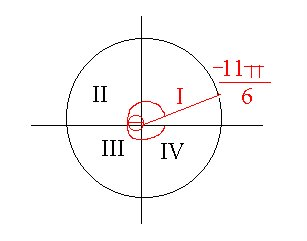4) Assuming the point P lies on the intersection of the unit circle and the line segment joining the origin to the point Q(-9,40), find the coordinates of P. (4 marks)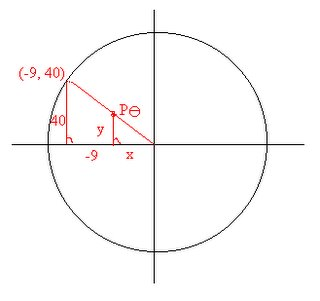(-9)2 +(40)2 = r2
81 + 1600 = root of 1681
root of 1681 = 41
x/-9 = 1/41 => x = -9/41
y/40 = 1/41 => y = 40/41
Sinθ = 40/41
Cosθ = -9/41
P θ = (-9/41,40/41)

*Remember if you draw a diagram you must correctly place the angle in the right place on the circle. For this question you would have lost 1 mark. Another thing is to add the equal sign where needed because you will lose marks and to correctly place the x and y coordinates in the answer.

After the quiz he collected it and randomly gave us other people's quizes. He told us how to correct it and where we would lose the marks.
You would lose marks in the following:
- Not adding the degrees symbol
- Incorrectly placing an angle on a diagram
- Not placing the equal sign on your work
- Incorrectly placing the x and y coordinates in the answer for example if the answer was Pθ=(9,40) but you put Pθ = (40,9).

There's more but I can't remember them but you get the idea right? The setup should always be correct because it's not only about the answer but the steps you take to get to the answer is important too.

I'm not done yet so bare with me. Just before the bell rang Mr.K wrote on the board
5x - 3 = 4x + 16
5tanx - 3 = 4tanx + 16
tanx = 19

He then told us we were looking for the arc tangent of 19 and told us to punch in 2nd function tangent then 19 into our calculators. The answer became 86.7º which we round off to 87º. We know that 87º is in quadrant one but wait there's a second answer as well.
Remember that sinθ maximum is -1 and 1 and cosθ maximum is -1 and 1 as well but tanθ has no maximum. So there will always be two answers. To find the second answer remember that in Quadrant I, tanθ is positive but in Quadrant III tanθ is positive as well. So you would have to add 87º after Quad II which will look like this:
87º + 180º = 267º which brings you to Quad III.

So that's it for me. Yay! If there are any mistakes please comment okay because I'm still new to this and this is my first post. Bye for now!

Oh by the way the next scribe will be........ hellochipsahoy

September 14, 2006

4th Scribe Post

Hey what's up everyone!?! , Hi I'm Tennyson today is my turn of being the scriber. Today at school we were learning more about the Quadrants,circles,and little bit of trig. There was some questions in the morning that we had to complete. Some was a bit difficult to understand but we all got through it. so here it is:

Which Quardrant is each angle in

Q:
1.
a). 5π/3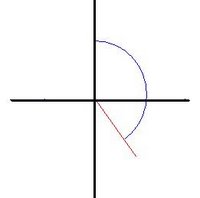How i got the answer was that i look at my pie plate. 5 π/3 is location in the plate at 300 degrees.

b).3 π/4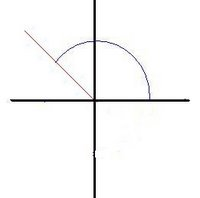When we know that 4 π/4 is a half the circle. So if think about it 3 π/4 is not just a half yet. So you see in my graph it shows. Also 3 π/4 is in your pie plate , located at 135 degrees.

c). 23 π/4

well π/4 is half a circle , to make it a full cirlce it would be 8 π/4. 3 rotation around would be 24 π/4. Right now we need it to be 23 π/4, so we know that it wouldn't be just exactly 24 π/4, so we rotation it backward a little so it would be in quadrant IV. Like in my diagram.

d). -41 π/6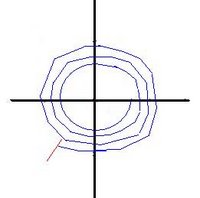Half circle would be - π/6, in order it to be full it would be -12 π/6. Goes around three times, it would equal to -48 π/6, we want it to be -41 π/6, so we go backward to equal -41. The differences between this question than the others is because of the negative , so when it rotates in a circle it goes the opposite direction.

2.
Q:Find P(θ) the coordintates of the point P on the unit circle that intersects A line drawn from the origin through the point (9,-40).

A:(9)2 +(-40)2 = r2
root of 1681
r= 41
y/-40 = 1/41 --> y= -40/41
x/9 = 1/41 -- > x= 9/41
P θ = (9/41,-40/41)

A:x2+x2 = (2)2
2(x)2/2 = 4/2
x= root 4
x=root 2

During class we were talking about , having θ is very important when it is in a sin,tan,or even cos. Like Without θ in the word sin, it would just be SIN , meaing doing something bad. So you have to have &theta right after.
Example : sinθ

The other thing we were talking about is the word "Invert".We know how to do it, but we don't know what it means.Invert is when your undoing your first answer into another answer that means the same. I know this might sound confusing here's a example:

sinθ=1/2
when you undo it, it would be:
sinθ-1 = 30 deg

How the answer is the same , is when you have:
sinθ30= 1/2